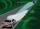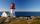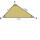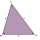# Right triangle + similarity of triangles - math problems

#### Number of problems found: 90

• Traffic lawsUnder traffic regulations, car lights can illuminate the road up to a maximum of 30 m. To check the reach of the dipped-beam lights of their car, Peter stopped the car at 1.5 m from the wall. The dipped beam headlights are 60 cm high. At what height on th
• MO Z7–I–6 2021In the triangle ABC, point D lies on the AC side and point E on the BC side. The sizes of the angles ABD, BAE, CAE and CBD are 30°, 60°, 20° and 30°, respectively. Find the size of the AED angle.
• Similarity of two trianglesThe KLM triangle has a side length of k = 6.3cm, l = 8.1cm, m = 11.1cm. The triangle XYZ has a side length of x = 8.4cm, y = 10.8cm, z = 14.8cm. Are triangle KLM and XYZ similar? (write 0 if not, if yes, find and write the coefficient of a similarity)
• Equilateral triangle ABCIn the equilateral triangle ABC, K is the center of the AB side, the L point lies on one-third of the BC side near the point C, and the point M lies in the one-third of the side of the AC side closer to the point A. Find what part of the ABC triangle cont
• Diagonals at right angleIn the trapezoid ABCD, this is given: AB=12cm CD=4cm And diagonals crossed under a right angle. What is the area of this trapezoid ABCD?
• Rectangular trapezoidThe ABCD rectangular trapezoid with the AB and CD bases is divided by the diagonal AC into two equilateral rectangular triangles. The length of the diagonal AC is 62cm. Calculate trapezium area in cm square and calculate how many differs perimeters of the
• Similarity coefficientIn the triangle TMA the length of the sides is t = 5cm, m = 3.5cm, a = 6.2cm. Another similar triangle has side lengths of 6.65 cm, 11.78 cm, 9.5 cm. Determine the similarity coefficient of these triangles and assign similar sides to each other.
• Tower's viewFrom the church tower's view at the height of 65 m, the top of the house can be seen at a depth angle of alpha = 45° and its bottom at a depth angle of beta = 58°. Calculate the height of the house and its distance from the church.
• LighthouseThe man, 180 cm tall, walks along the seafront directly to the lighthouse. The male shadow caused by the beacon light is initially 5.4 meters long. When the man approaches the lighthouse by 90 meters, its shadow shorter by 3 meters. How tall is the lighthMast has 13 m long shadow on a slope rising from the mast foot in the direction of the shadow angle at angle 15°. Determine the height of the mast, if the sun above the horizon is at angle 33°. Use the law of sines.
• LighthouseMarcel (point J) lies in the grass and sees the top of the tent (point T) and behind it the top of the lighthouse (P). | TT '| = 1.2m, | PP '| = 36m, | JT '| = 5m. Marcel lies 15 meters away from the sea (M). Calculate the lighthouse distance from the sea
• Triangle KLBIt is given equilateral triangle ABC. From point L which is the midpoint of the side BC of the triangle it is drwn perpendicular to the side AB. Intersection of perpendicular and the side AB is point K. How many % of the area of the triangle ABC is area oMiro stands under a tree and watching its shadow and shadow of the tree. Miro is 180 cm tall and its shade is 1.5 m long. The shadow of the tree is three times as long as Miro's shadow. How tall is the tree in meters?
• MO - trianglesOn the AB and AC sides of the triangle ABC lies successive points E and F, on segment EF lie point D. The EF and BC lines are parallel and is true this ratio FD:DE = AE:EB = 2:1. The area of ABC triangle is 27 hectares and line segments EF, AD, and DB seg
• Reverse Pythagorean theoremGiven are lengths of the sides of the triangles. Decide which one is rectangular: Δ ABC: 77 dm, 85 dm, 36 dm ... Δ DEF: 55 dm, 82 dm, 61 dm ... Δ GHI: 24 mm, 25 mm, 7 mm ... Δ JKL: 32 dm, 51 dm, 82 dm ... Δ MNO: 51 dm, 45 dm,
• Similarity coefficientThe triangles ABC and A "B" C "are similar to the similarity coefficient 2. The sizes of the angles of the triangle ABC are α = 35° and β = 48°. Find the magnitudes of all angles of triangle A "B" C ".
• Similarity coefficientThe ratio of similarity of two equilateral triangles is 3.5 (ie 7:2). The length of the side of smaller triangle is 2.4 cm. Calculate the perimeter and area of ​​the larger triangle.
• Vertical rodThe vertical one-meter-long rod casts a shadow 150 cm long. Calculate the height of a column whose shadow is 36 m long at the same time.
• ThereThere is a stretched steel cable between the 3 columns. The height of the first column is 4 m, the height of the second is 3.5 m. The distance between the first two columns is 2.5 m, the distance between the second and third is 5 m. The heels of all threeTree perpendicular to the horizontal surface has a shadow 8.32 meters long. At the same time meter rod perpendicular to the horizontal surface has shadow 64 cm long. How tall is tree?